## 3.3 The Atom and Atomic Theory

### Learning Objectives

By the end of this section, you will be able to:

• Outline milestones in the development of modern atomic theory
• Summarize and interpret the results of the experiments of Thomson, Millikan, and Rutherford
• Describe the three subatomic particles that compose atoms
• Define isotopes and give examples for several elements
• Write isotopic symbols for elements and ions.

The smallest piece of an element that maintains the identity of that element is called an atom. Individual atoms are extremely small. It would take about fifty million atoms in a row to make a line that is 1 cm long. The period at the end of a printed sentence has several million atoms in it. Atoms are so small that it is difficult to believe that all matter is made from atoms—but it is.

The concept that atoms play a fundamental role in chemistry is formalized by the modern atomic theory, first stated by John Dalton, an English scientist, in 1808. It consists of three parts:

1. All matter is composed of atoms.
2. Atoms of the same element are the same; atoms of different elements are different.
3. Atoms combine in whole-number ratios to form compounds.

These concepts form the basis of chemistry.

In the two centuries since Dalton developed his ideas, scientists have made significant progress in furthering our understanding of atomic theory. Much of this came from the results of several seminal experiments that revealed the details of the internal structure of atoms. Here, we will discuss some of those key developments, with an emphasis on application of the scientific method, as well as understanding how the experimental evidence was analyzed. While the historical persons and dates behind these experiments can be quite interesting, it is most important to understand the concepts resulting from their work.

## Evolution of the Atom

If matter were composed of atoms, what were atoms composed of? Were they the smallest particles, or was there something smaller? In the late 1800s, a number of scientists interested in questions like these investigated the electrical discharges that could be produced in low-pressure gases, with the most significant discovery made by English physicist J. J. Thomson using a cathode ray tube. This apparatus consisted of a sealed glass tube from which almost all the air had been removed; the tube contained two metal electrodes. When high voltage was applied across the electrodes, a visible beam called a cathode ray appeared between them. This beam was deflected toward the positive charge and away from the negative charge, and was produced in the same way with identical properties when different metals were used for the electrodes. In similar experiments, the ray was simultaneously deflected by an applied magnetic field, and measurements of the extent of deflection and the magnetic field strength allowed Thomson to calculate the charge-to-mass ratio of the cathode ray particles. The results of these measurements indicated that these particles were much lighter than atoms (Figure 1).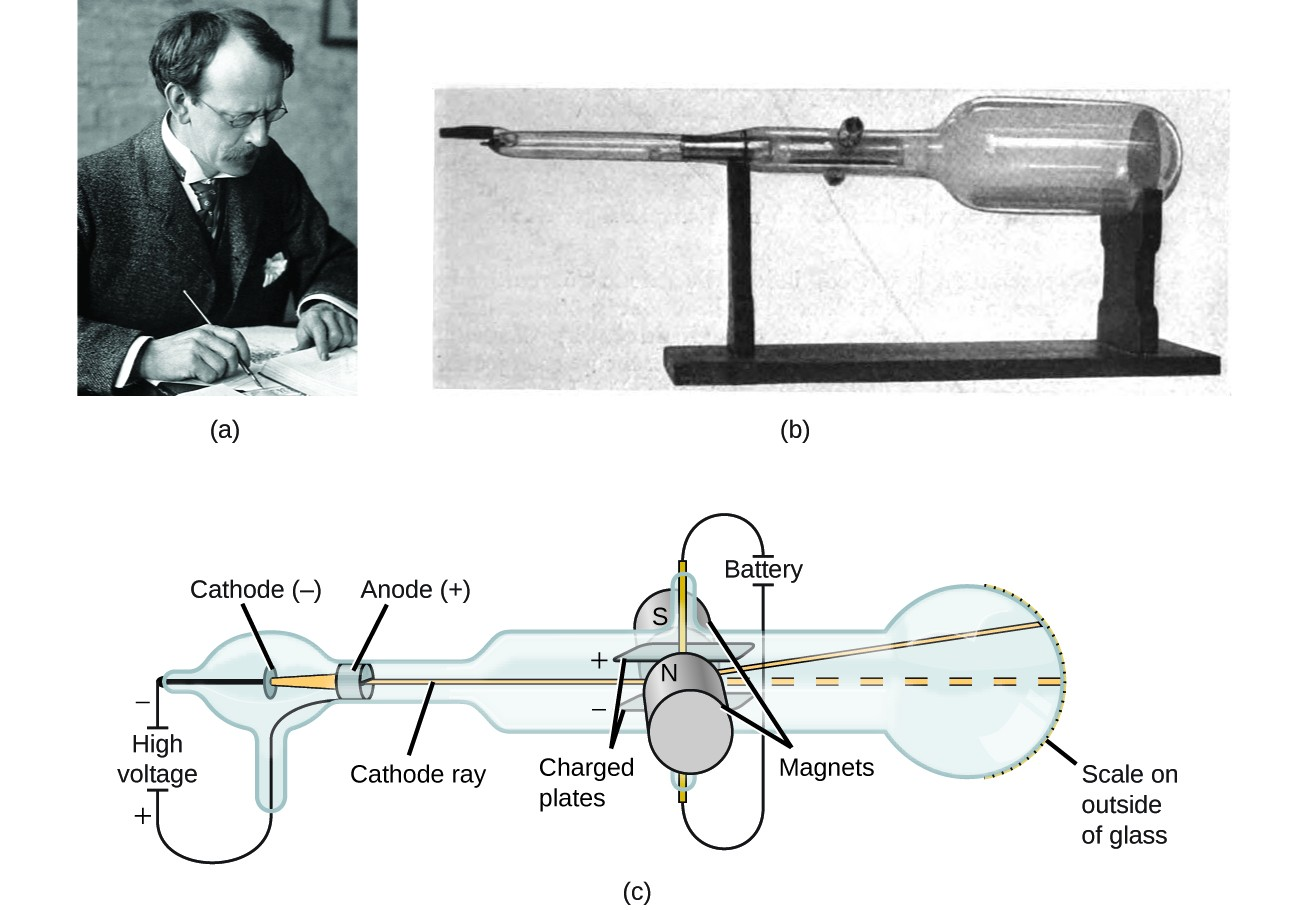Figure 1. (a) J. J. Thomson produced a visible beam in a cathode ray tube. (b) This is an early cathode ray tube, invented in 1897 by Ferdinand Braun. (c) In the cathode ray, the beam (shown in yellow) comes from the cathode and is accelerated past the anode toward a fluorescent scale at the end of the tube. Simultaneous deflections by applied electric and magnetic fields permitted Thomson to calculate the mass-to-charge ratio of the particles composing the cathode ray. (credit a: modification of work by Nobel Foundation; credit b: modification of work by Eugen Nesper; credit c: modification of work by “Kurzon”/Wikimedia Commons)

Based on his observations, here is what Thomson proposed and why: The particles are attracted by positive (+) charges and repelled by negative (-) charges, so they must be negatively charged (like charges repel and unlike charges attract); they are less massive than atoms and indistinguishable, regardless of the source material, so they must be fundamental, subatomic constituents of all atoms. Although controversial at the time, Thomson’s idea was gradually accepted, and his cathode ray particle is what we now call an electron, a negatively charged, subatomic particle with a mass more than one thousand-times less that of an atom. The term “electron” was coined in 1891 by Irish physicist George Stoney, from “electric ion.”

Click this link to “JJ Thompson Talks About the Size of the Electron” to hear Thomson describe his discovery in his own voice.

Scientists had now established that the atom was not indivisible as Dalton had believed, and due to the work of Thomson and others, the charge and mass of the negative, subatomic particles—the electrons—were known. However, the positively charged part of an atom was not yet well understood. In 1904, Thomson proposed the “plum pudding” model of atoms, which described a positively charged mass with an equal amount of negative charge in the form of electrons embedded in it, since all atoms are electrically neutral. A competing model had been proposed in 1903 by Hantaro Nagaoka, who postulated a Saturn-like atom, consisting of a positively charged sphere surrounded by a halo of electrons (Figure 2).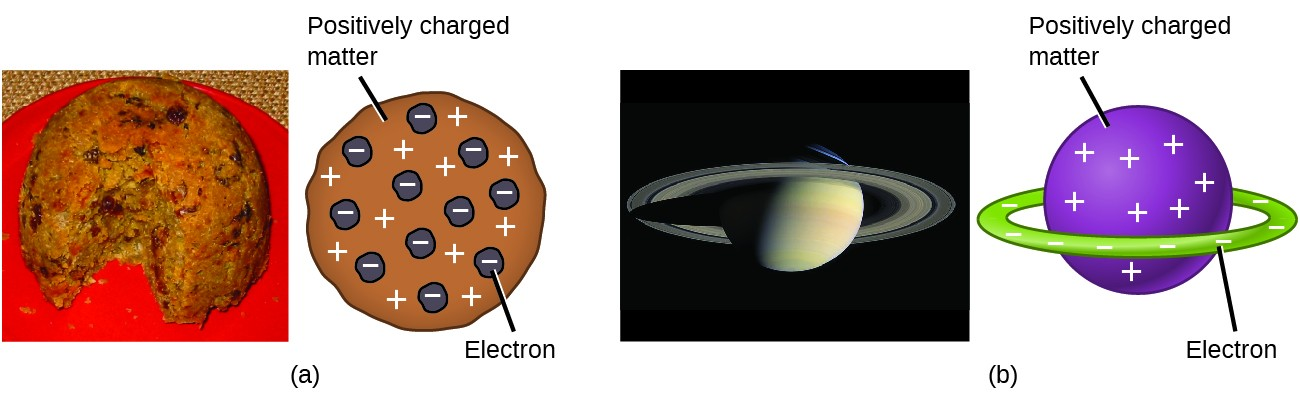Figure 2. (a) Thomson suggested that atoms resembled plum pudding, an English dessert consisting of moist cake with embedded raisins (“plums”). (b) Nagaoka proposed that atoms resembled the planet Saturn, with a ring of electrons surrounding a positive “planet.” (credit a: modification of work by “Man vyi”/Wikimedia Commons; credit b: modification of work by “NASA”/Wikimedia Commons)

The next major development in understanding the atom came from Ernest Rutherford, a physicist from New Zealand who largely spent his scientific career in Canada and England. He performed a series of experiments using a beam of high-speed, positively charged alpha particles (α particles) that were produced by the radioactive decay of radium; α particles consist of two protons and two neutrons (you will learn more about radioactive decay in the chapter on nuclear chemistry). Rutherford and his colleagues Hans Geiger (later famous for the Geiger counter) and Ernest Marsden aimed a beam of α particles, the source of which was embedded in a lead block to absorb most of the radiation, at a very thin piece of gold foil and examined the resultant scattering of the α particles using a luminescent screen that glowed briefly where hit by an α particle.

What did they discover? Most particles passed right through the foil without being deflected at all. However, some were diverted slightly, and a very small number were deflected almost straight back toward the source (Figure 3). Rutherford described finding these results: “It was quite the most incredible event that has ever happened to me in my life. It was almost as incredible as if you fired a 15-inch shell at a piece of tissue paper and it came back and hit you.”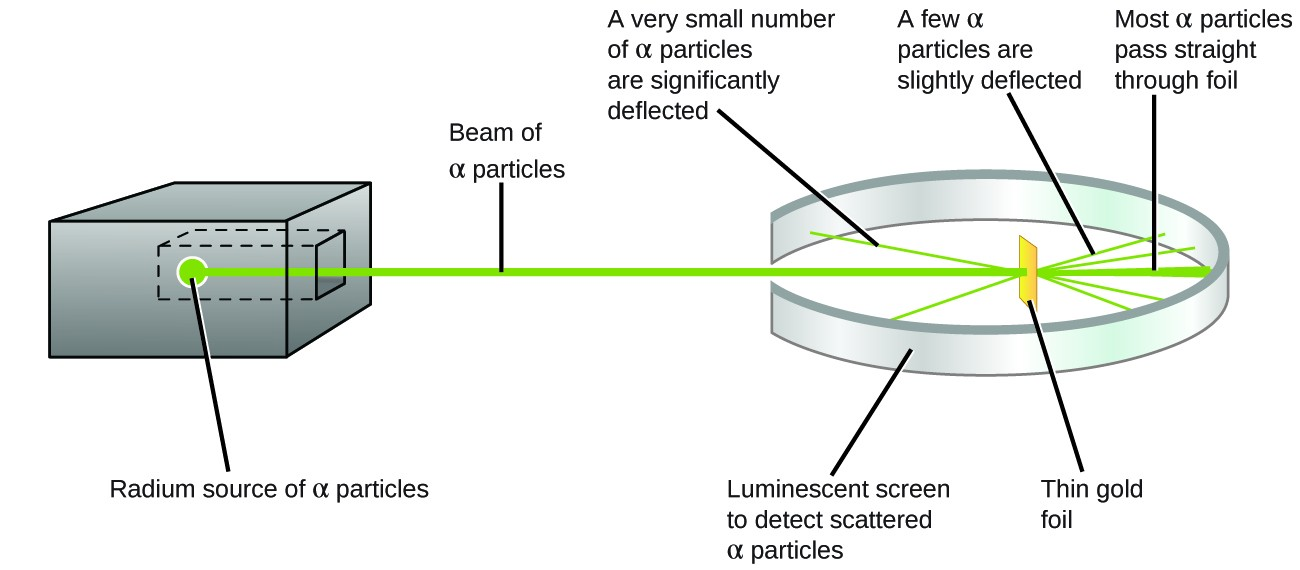Figure 3. Geiger and Rutherford fired α particles at a piece of gold foil and detected where those particles went, as shown in this schematic diagram of their experiment. Most of the particles passed straight through the foil, but a few were deflected slightly and a very small number were significantly deflected.

Here is what Rutherford deduced: Because most of the fast-moving α particles passed through the gold atoms undeflected, they must have traveled through essentially empty space inside the atom. Alpha particles are positively charged, so deflections arose when they encountered another positive charge (like charges repel each other). Since like charges repel one another, the few positively charged α particles that changed paths abruptly must have hit, or closely approached, another body that also had a highly concentrated, positive charge. Since the deflections occurred a small fraction of the time, this charge only occupied a small amount of the space in the gold foil. Analyzing a series of such experiments in detail, Rutherford drew two conclusions:

1. The volume occupied by an atom must consist of a large amount of empty space.
2. A small, relatively heavy, positively charged body, the nucleus, must be at the center of each atom.
View this simulation of the Rutherford gold foil experiment. Adjust the slit width to produce a narrower or broader beam of α particles to see how that affects the scattering pattern.

This analysis led Rutherford to propose a model in which an atom consists of a very small, positively charged nucleus, in which most of the mass of the atom is concentrated, surrounded by the negatively charged electrons, so that the atom is electrically neutral (Figure 4). After many more experiments, Rutherford also discovered that the nuclei of other elements contain the hydrogen nucleus as a “building block,” and he named this more fundamental particle the proton, the positively charged, subatomic particle found in the nucleus. With one addition, which you will learn next, this nuclear model of the atom, proposed over a century ago, is still used today.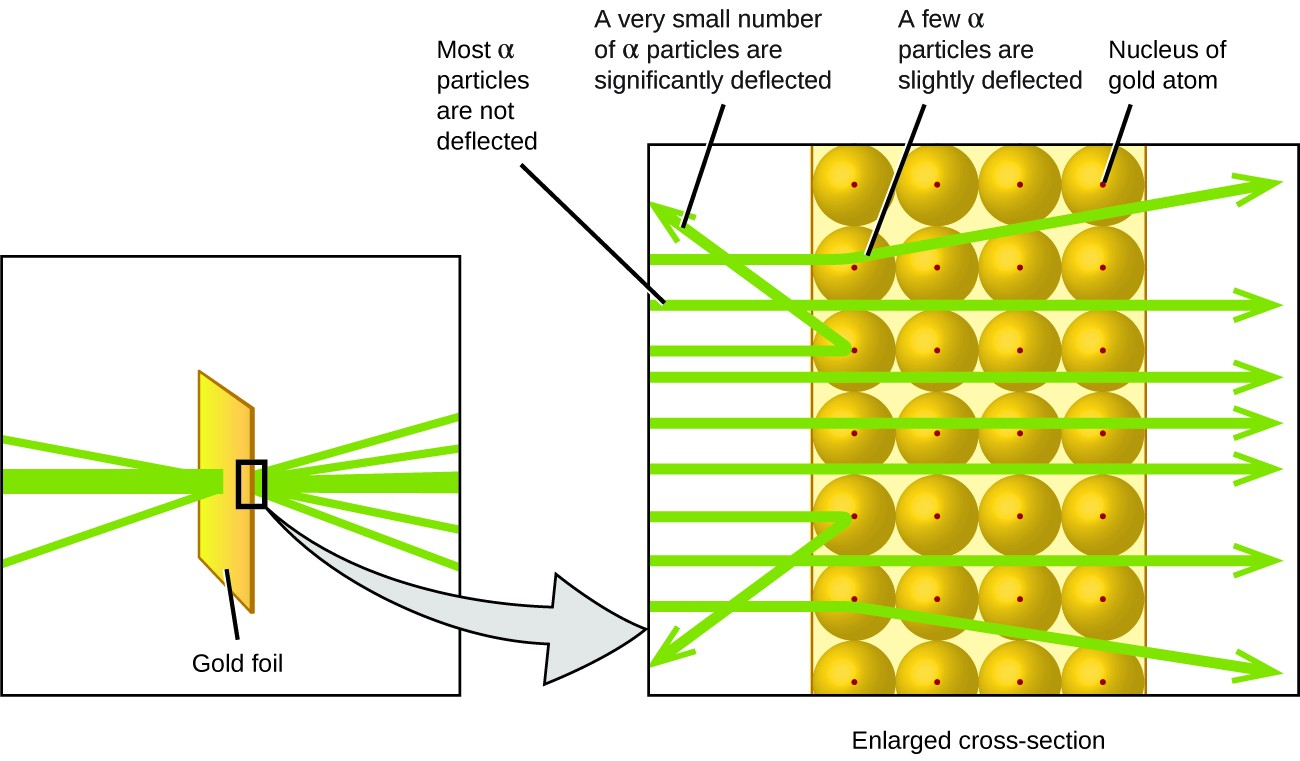Figure 4. The α particles are deflected only when they collide with or pass close to the much heavier, positively charged gold nucleus. Because the nucleus is very small compared to the size of an atom, very few α particles are deflected. Most pass through the relatively large region occupied by electrons, which are too light to deflect the rapidly moving particles.

The Rutherford Scattering simulation allows you to investigate the differences between a “plum pudding” atom and a Rutherford atom by firing α particles at each type of atom.

Another important finding was the discovery of isotopes. During the early 1900s, scientists identified several substances that appeared to be new elements, isolating them from radioactive ores. For example, a “new element” produced by the radioactive decay of thorium was initially given the name mesothorium. However, a more detailed analysis showed that mesothorium was chemically identical to radium (another decay product), despite having a different atomic mass. This result, along with similar findings for other elements, led the English chemist Frederick Soddy to realize that an element could have types of atoms with different masses that were chemically indistinguishable. These different types are called isotopes—atoms of the same element that differ in mass. Soddy was awarded the Nobel Prize in Chemistry in 1921 for this discovery.

One puzzle remained: The nucleus was known to contain almost all of the mass of an atom, with the number of protons only providing half, or less, of that mass. Different proposals were made to explain what constituted the remaining mass, including the existence of neutral particles in the nucleus. As you might expect, detecting uncharged particles is very challenging, and it was not until 1932 that James Chadwick found evidence of neutrons, uncharged, subatomic particles with a mass approximately the same as that of protons. The existence of the neutron also explained isotopes: They differ in mass because they have different numbers of neutrons, but they are chemically identical because they have the same number of protons. With the discovery of the neutron, the modern model of an atom was established (Figure 5).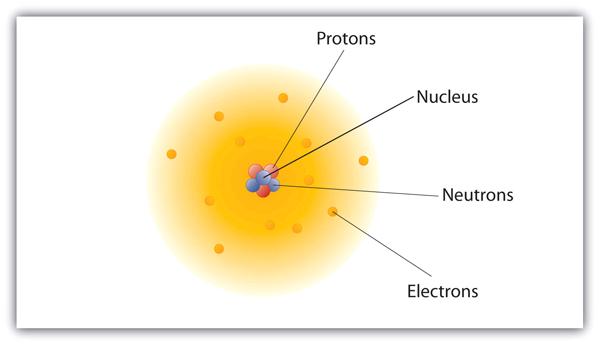Figure 5. The structure of an atom. The nucleus of an atom is composed of protons and neutrons. The nucleus is surrounded by an electron cloud.

The diameter of an atom is on the order of 10−10 m, whereas the diameter of the nucleus is roughly 10−15 m—about 100,000 times smaller. For a perspective about their relative sizes, consider this: If the nucleus were the size of a blueberry, the atom would be about the size of a football stadium (Figure 6).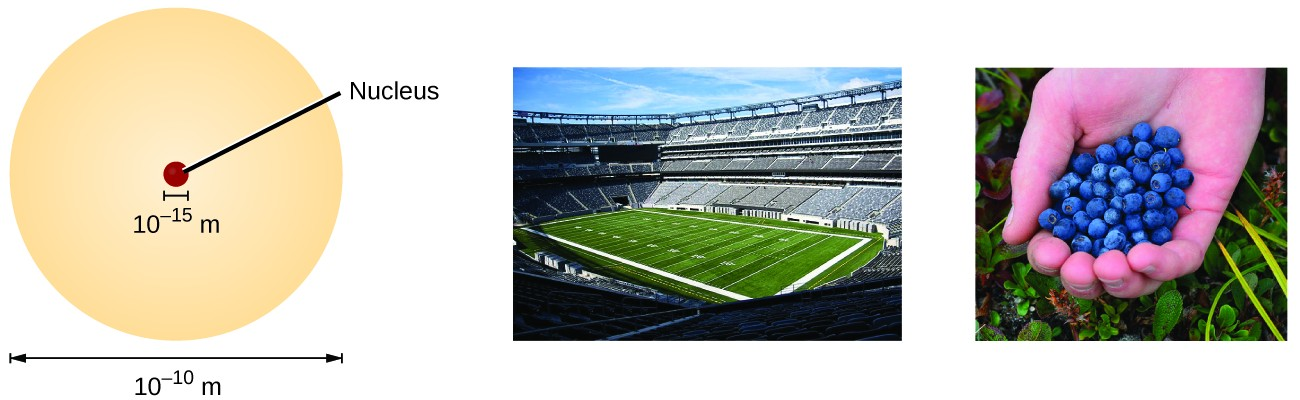Figure 6. If an atom could be expanded to the size of a football stadium, the nucleus would be the size of a single blueberry. (credit middle: modification of work by “babyknight”/Wikimedia Commons; credit right: modification of work by Paxson Woelber)

Atoms—and the protons, neutrons, and electrons that compose them—are extremely small. For example, a carbon atom weighs less than 2 × 10−23 g, and an electron has a charge of less than 2 × 10−19 C (coulomb). When describing the properties of tiny objects such as atoms, we use appropriately small units of measure, such as the atomic mass unit (amu) and the fundamental unit of charge (e). The amu was originally defined based on hydrogen, the lightest element, then later in terms of oxygen. Since 1961, it has been defined with regard to the most abundant isotope of carbon, atoms of which are assigned masses of exactly 12 amu. (This isotope is known as “carbon-12” as will be discussed later in this module.) Thus, one amu is exactly $\frac{1}{12}$ of the mass of one carbon-12 atom: 1 amu = 1.6605 × 10−24 g. (The Dalton (Da) and the unified atomic mass unit (u) are alternative units that are equivalent to the amu.) The fundamental unit of charge (also called the elementary charge) equals the magnitude of the charge of an electron (e) with e = 1.602 × 10−19 C.

A proton has a mass of 1.0073 amu and a charge of 1+. A neutron is a slightly heavier particle with a mass 1.0087 amu and a charge of zero; as its name suggests, it is neutral. The electron has a charge of 1− and is a much lighter particle with a mass of about 0.00055 amu (it would take about 1800 electrons to equal the mass of one proton. The properties of these fundamental particles are summarized in Table 1. (An observant student might notice that the sum of an atom’s subatomic particles does not equal the atom’s actual mass: The total mass of six protons, six neutrons, and six electrons is 12.0993 amu, slightly larger than 12.00 amu. This “missing” mass is known as the mass defect, and you will learn about it in the chapter on nuclear chemistry.)

Table 1. Properties of Subatomic Particles
Name Location Charge (C) Unit Charge Mass (amu) Mass (g)
electron outside nucleus −1.602 × 10−19 1− 0.00055 0.00091 × 10−24
proton nucleus 1.602 × 10−19 1+ 1.00727 1.67262 × 10−24
neutron nucleus 0 0 1.00866 1.67493 × 10−24

The number of protons in the nucleus of an atom is its atomic number (Z). This is the defining trait of an element: Its value determines the identity of the atom. For example, any atom that contains six protons is the element carbon and has the atomic number 6, regardless of how many neutrons or electrons it may have. A neutral atom must contain the same number of positive and negative charges, so the number of protons equals the number of electrons. Therefore, the atomic number also indicates the number of electrons in an atom. The total number of protons and neutrons in an atom is called its mass number (A). The number of neutrons is therefore the difference between the mass number and the atomic number: A – Z = number of neutrons.

$\begin{array}{ccc}\hfill \text{atomic number}\left(\text{Z}\right)& =& \text{number of protons}\hfill \\ \hfill \text{atomic mass}\left(\text{A}\right)& =& \text{number of protons}+\text{number of neutrons}\hfill \\ \hfill \text{A}-\text{Z}& =& \text{number of neutrons}\hfill \end{array}$

Atoms are electrically neutral if they contain the same number of positively charged protons and negatively charged electrons. When the numbers of these subatomic particles are not equal, the atom is electrically charged and is called an ion. The charge of an atom is defined as follows:

Atomic charge = number of protons − number of electrons

As will be discussed in more detail later in this chapter, atoms (and molecules) typically acquire charge by gaining or losing electrons. An atom that gains one or more electrons will exhibit a negative charge and is called an anion. Positively charged atoms called cations are formed when an atom loses one or more electrons. For example, a neutral sodium atom (Z = 11) has 11 electrons. If this atom loses one electron, it will become a cation with a 1+ charge (11 − 10 = 1+). A neutral oxygen atom (Z = 8) has eight electrons, and if it gains two electrons it will become an anion with a 2− charge (8 − 10 = 2−).

### Example 1: Composition of an Atom

Iodine is an essential trace element in our diet; it is needed to produce thyroid hormone. Insufficient iodine in the diet can lead to the development of a goiter, an enlargement of the thyroid gland (Figure 2).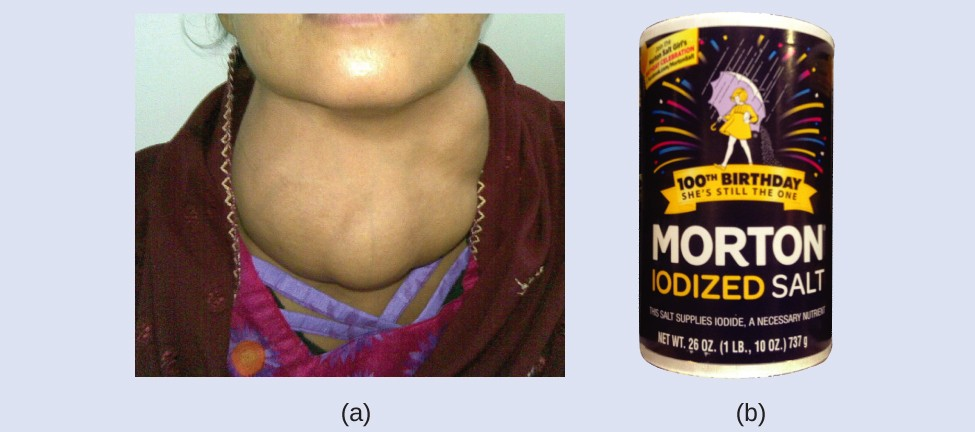Figure 2. (a) Insufficient iodine in the diet can cause an enlargement of the thyroid gland called a goiter. (b) The addition of small amounts of iodine to salt, which prevents the formation of goiters, has helped eliminate this concern in the US where salt consumption is high. (credit a: modification of work by “Almazi”/Wikimedia Commons; credit b: modification of work by Mike Mozart)

The addition of small amounts of iodine to table salt (iodized salt) has essentially eliminated this health concern in the United States, but as much as 40% of the world’s population is still at risk of iodine deficiency. The iodine atoms are added as anions, and each has a 1− charge and a mass number of 127. Determine the numbers of protons, neutrons, and electrons in one of these iodine anions.

An atom of platinum has a mass number of 195 and contains 74 electrons. How many protons and neutrons does it contain, and what is its charge?

## Chemical Symbols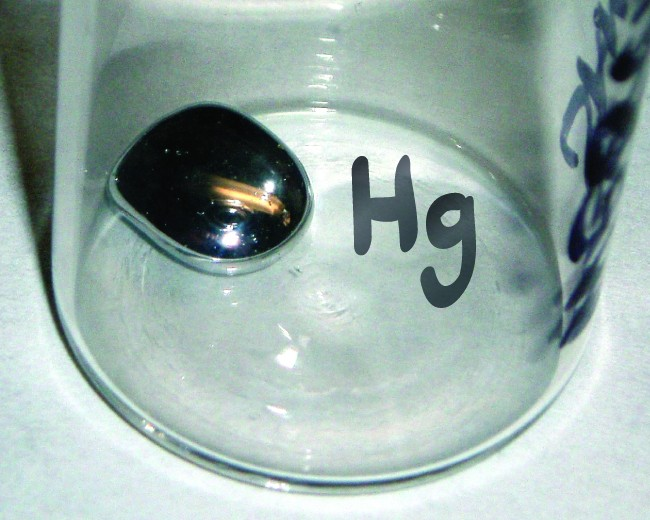Figure 7. The symbol Hg represents the element mercury regardless of the amount; it could represent one atom of mercury or a large amount of mercury.

A chemical symbol is an abbreviation that we use to indicate an element or an atom of an element. For example, the symbol for mercury is Hg (Figure 7). We use the same symbol to indicate one atom of mercury (microscopic domain) or to label a container of many atoms of the element mercury (macroscopic domain).

The symbols for several common elements and their atoms are listed in Table 2. Some symbols are derived from the common name of the element; others are abbreviations of the name in another language. Most symbols have one or two letters, but three-letter symbols have been used to describe some elements that have atomic numbers greater than 112.

To avoid confusion with other notations, only the first letter of a symbol is capitalized. For example, Co is the symbol for the element cobalt, but CO is the notation for the compound carbon monoxide, which contains atoms of the elements carbon (C) and oxygen (O). All known elements and their symbols are in the periodic table.

Table 2. Some Common Elements and Their Symbols
Element Symbol Element Symbol
aluminum Al iron Fe (from ferrum)
bromine Br lead Pb (from plumbum)
calcium Ca magnesium Mg
carbon C mercury Hg (from hydrargyrum)
chlorine Cl nitrogen N
chromium Cr oxygen O
cobalt Co potassium K (from kalium)
copper Cu (from cuprum) silicon Si
fluorine F silver Ag (from argentum)
gold Au (from aurum) sodium Na (from natrium)
helium He sulfur S
hydrogen H tin Sn (from stannum)
iodine I zinc Zn

Traditionally, the discoverer (or discoverers) of a new element names the element. However, until the name is recognized by the International Union of Pure and Applied Chemistry (IUPAC), the recommended name of the new element is based on the Latin word(s) for its atomic number. For example, element 106 was called unnilhexium (Unh), element 107 was called unnilseptium (Uns), and element 108 was called unniloctium (Uno) for several years. These elements are now named after scientists (or occasionally locations); for example, element 106 is now known as seaborgium (Sg) in honor of Glenn Seaborg, a Nobel Prize winner who was active in the discovery of several heavy elements.

## Isotopes

The symbol for a specific isotope of any element is written by placing the mass number as a superscript to the left of the element symbol (Figure 8). The atomic number is sometimes written as a subscript preceding the symbol, but since this number defines the element’s identity, as does its symbol, it is often omitted. For example, magnesium exists as a mixture of three isotopes, each with an atomic number of 12 and with mass numbers of 24, 25, and 26, respectively. These isotopes can be identified as 24Mg, 25Mg, and 26Mg. These isotope symbols are read as “element, mass number” and can be symbolized consistent with this reading. For instance, 24Mg is read as “magnesium 24,” and can be written as “magnesium-24” or “Mg-24.” 25Mg is read as “magnesium 25,” and can be written as “magnesium-25” or “Mg-25.” All magnesium atoms have 12 protons in their nucleus. They differ only because a 24Mg atom has 12 neutrons in its nucleus, a 25Mg atom has 13 neutrons, and a 26Mg has 14 neutrons.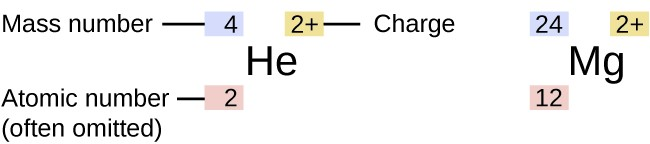Figure 8. The symbol for an atom indicates the element via its usual two-letter symbol, the mass number as a left superscript, the atomic number as a left subscript (sometimes omitted), and the charge as a right superscript.

Information about the naturally occurring isotopes of elements with atomic numbers 1 through 10 is given in Table 3. Note that in addition to standard names and symbols, the isotopes of hydrogen are often referred to using common names and accompanying symbols. Hydrogen-2, symbolized 2H, is also called deuterium and sometimes symbolized D. Hydrogen-3, symbolized 3H, is also called tritium and sometimes symbolized T.

Table 3. Nuclear Compositions of Atoms of the Very Light Elements
Element Symbol Atomic Number Number of Protons Number of Neutrons Mass (amu) % Natural Abundance
hydrogen ${}_{1}^{1}\text{H}$
(protium)
1 1 0 1.0078 99.989
${}_{1}^{2}\text{H}$
(deuterium)
1 1 1 2.0141 0.0115
${}_{1}^{3}\text{H}$
(tritium)
1 1 2 3.01605 — (trace)
helium ${}_{2}^{3}\text{He}$ 2 2 1 3.01603 0.00013
${}_{2}^{4}\text{He}$ 2 2 2 4.0026 100
lithium ${}_{3}^{6}\text{Li}$ 3 3 3 6.0151 7.59
${}_{3}^{7}\text{Li}$ 3 3 4 7.0160 92.41
beryllium ${}_{4}^{9}\text{Be}$ 4 4 5 9.0122 100
boron ${}_{5}^{10}\text{B}$ 5 5 5 10.0129 19.9
${}_{5}^{11}\text{B}$ 5 5 6 11.0093 80.1
carbon ${}_{6}^{12}\text{C}$ 6 6 6 12.0000 98.89
${}_{6}^{13}\text{C}$ 6 6 7 13.0034 1.11
${}_{6}^{14}\text{C}$ 6 6 8 14.0032 — (trace)
nitrogen ${}_{7}^{14}\text{N}$ 7 7 7 14.0031 99.63
${}_{7}^{15}\text{N}$ 7 7 8 15.0001 0.37
oxygen ${}_{8}^{16}\text{O}$ 8 8 8 15.9949 99.757
${}_{8}^{17}\text{O}$ 8 8 9 16.9991 0.038
${}_{8}^{18}\text{O}$ 8 8 10 17.9992 0.205
fluorine ${}_{9}^{19}\text{F}$ 9 9 10 18.9984 100
neon ${}_{10}^{20}\text{Ne}$ 10 10 10 19.9924 90.48
${}_{10}^{21}\text{Ne}$ 10 10 11 20.9938 0.27
${}_{10}^{22}\text{Ne}$ 10 10 12 21.9914 9.25
Use this Build an Atom simulator to build atoms of the first 10 elements, see which isotopes exist, check nuclear stability, and gain experience with isotope symbols.

## Atomic Mass

Because each proton and each neutron contribute approximately one amu to the mass of an atom, and each electron contributes far less, the atomic mass of a single atom is approximately equal to its mass number (a whole number). However, the average masses of atoms of most elements are not whole numbers because most elements exist naturally as mixtures of two or more isotopes.

The mass of an element shown in a periodic table or listed in a table of atomic masses is a weighted, average mass of all the isotopes present in a naturally occurring sample of that element. This is equal to the sum of each individual isotope’s mass multiplied by its fractional abundance.

$\text{average mass}=\sum _{i}{\left(\text{fractional abundance}\times \text{isotopic mass}\right)}_{i}$

For example, the element boron is composed of two isotopes: About 19.9% of all boron atoms are 10B with a mass of 10.0129 amu, and the remaining 80.1% are 11B with a mass of 11.0093 amu. The average atomic mass for boron is calculated to be:

$\begin{array}{cc}\hfill \text{boron average mass}& =\left(0.199\times \text{10.0129 amu}\right)+\left(0.801\times \text{11.0093 amu}\right)\hfill \\ & =\text{1.99 amu}+\text{8.82 amu}\hfill \\ & =\text{10.81 amu}\hfill \end{array}$

It is important to understand that no single boron atom weighs exactly 10.8 amu; 10.8 amu is the average mass of all boron atoms, and individual boron atoms weigh either approximately 10 amu or 11 amu.

### Example 2: Calculation of Average Atomic Mass

A meteorite found in central Indiana contains traces of the noble gas neon picked up from the solar wind during the meteorite’s trip through the solar system. Analysis of a sample of the gas showed that it consisted of 91.84% 20Ne (mass 19.9924 amu), 0.47% 21Ne (mass 20.9940 amu), and 7.69% 22Ne (mass 21.9914 amu). What is the average mass of the neon in the solar wind?

A sample of magnesium is found to contain 78.70% of 24Mg atoms (mass 23.98 amu), 10.13% of 25Mg atoms (mass 24.99 amu), and 11.17% of 26Mg atoms (mass 25.98 amu). Calculate the average mass of a Mg atom.

Visit the PhET Isotopes and Atomic Mass site to make mixtures of the main isotopes of the first 18 elements, gain experience with average atomic mass, and check naturally occurring isotope ratios using the Isotopes and Atomic Mass simulation.

### Key Concepts and Summary

Although no one has actually seen the inside of an atom, experiments have demonstrated much about atomic structure. Thomson’s cathode ray tube showed that atoms contain small, negatively charged particles called electrons. Millikan discovered that there is a fundamental electric charge—the charge of an electron. Rutherford’s gold foil experiment showed that atoms have a small, dense, positively charged nucleus; the positively charged particles within the nucleus are called protons. Chadwick discovered that the nucleus also contains neutral particles called neutrons. Soddy demonstrated that atoms of the same element can differ in mass; these are called isotopes.

An atom consists of a small, positively charged nucleus surrounded by electrons. The nucleus contains protons and neutrons; its diameter is about 100,000 times smaller than that of the atom. The mass of one atom is usually expressed in atomic mass units (amu), which is referred to as the atomic mass. An amu is defined as exactly $\frac{1}{12}$ of the mass of a carbon-12 atom and is equal to 1.6605 × 10−24 g.

Protons are relatively heavy particles with a charge of 1+ and a mass of 1.0073 amu. Neutrons are relatively heavy particles with no charge and a mass of 1.0087 amu. Electrons are light particles with a charge of 1− and a mass of 0.00055 amu. The number of protons in the nucleus is called the atomic number (Z) and is the property that defines an atom’s elemental identity. The sum of the numbers of protons and neutrons in the nucleus is called the mass number and, expressed in amu, is approximately equal to the mass of the atom. An atom is neutral when it contains equal numbers of electrons and protons.

Isotopes of an element are atoms with the same atomic number but different mass numbers; isotopes of an element, therefore, differ from each other only in the number of neutrons within the nucleus. When a naturally occurring element is composed of several isotopes, the atomic mass of the element represents the average of the masses of the isotopes involved. A chemical symbol identifies the atoms in a substance using symbols, which are one-, two-, or three-letter abbreviations for the atoms.

#### Key Equations

• $\text{average mass}=\sum _{i}{\left(\text{fractional abundance}\times \text{isotopic mass}\right)}_{i}$

### Exercises

1. The existence of isotopes violates one of the original ideas of Dalton’s atomic theory. Which one?
2. How are electrons and protons similar? How are they different?
3. How are protons and neutrons similar? How are they different?
4. Predict and test the behavior of α particles fired at a “plum pudding” model atom.
1. Predict the paths taken by α particles that are fired at atoms with a Thomson’s plum pudding model structure. Explain why you expect the α particles to take these paths.
2. If α particles of higher energy than those in (a) are fired at plum pudding atoms, predict how their paths will differ from the lower-energy α particle paths. Explain your reasoning.
3. Now test your predictions from (a) and (b). Open the Rutherford Scattering simulation and select the “Plum Pudding Atom” tab. Set “Alpha Particles Energy” to “min,” and select “show traces.” Click on the gun to start firing α particles. Does this match your prediction from (a)? If not, explain why the actual path would be that shown in the simulation. Hit the pause button, or “Reset All.” Set “Alpha Particles Energy” to “max,” and start firing α particles. Does this match your prediction from (b)? If not, explain the effect of increased energy on the actual paths as shown in the simulation.
5. Predict and test the behavior of α particles fired at a Rutherford atom model.
1. Predict the paths taken by α particles that are fired at atoms with a Rutherford atom model structure. Explain why you expect the α particles to take these paths.
2. If α particles of higher energy than those in (a) are fired at Rutherford atoms, predict how their paths will differ from the lower-energy α particle paths. Explain your reasoning.
3. Predict how the paths taken by the α particles will differ if they are fired at Rutherford atoms of elements other than gold. What factor do you expect to cause this difference in paths, and why?
4. Now test your predictions from (a), (b), and (c). Open the Rutherford Scattering simulation and select the “Rutherford Atom” tab. Due to the scale of the simulation, it is best to start with a small nucleus, so select “20” for both protons and neutrons, “min” for energy, show traces, and then start firing α particles. Does this match your prediction from (a)? If not, explain why the actual path would be that shown in the simulation. Pause or reset, set energy to “max,” and start firing α particles. Does this match your prediction from (b)? If not, explain the effect of increased energy on the actual path as shown in the simulation. Pause or reset, select “40” for both protons and neutrons, “min” for energy, show traces, and fire away. Does this match your prediction from (c)? If not, explain why the actual path would be that shown in the simulation. Repeat this with larger numbers of protons and neutrons. What generalization can you make regarding the type of atom and effect on the path of α particles? Be clear and specific.
6. In what way are isotopes of a given element always different? In what way(s) are they always the same?
7. Write the symbol for each of the following ions:
1. the ion with a 1+ charge, atomic number 55, and mass number 133
2. the ion with 54 electrons, 53 protons, and 74 neutrons
3. the ion with atomic number 15, mass number 31, and a 3− charge
4. the ion with 24 electrons, 30 neutrons, and a 3+ charge
8. Write the symbol for each of the following ions:
1. the ion with a 3+ charge, 28 electrons, and a mass number of 71
2. the ion with 36 electrons, 35 protons, and 45 neutrons
3. the ion with 86 electrons, 142 neutrons, and a 4+ charge
4. the ion with a 2+ charge, atomic number 38, and mass number 87
9. Open the Build an Atom simulation and click on the Atom icon.
1. Pick any one of the first 10 elements that you would like to build and state its symbol.
2. Drag protons, neutrons, and electrons onto the atom template to make an atom of your element. State the numbers of protons, neutrons, and electrons in your atom, as well as the net charge and mass number.
3. Click on “Net Charge” and “Mass Number,” check your answers to (b), and correct, if needed.
4. Predict whether your atom will be stable or unstable. State your reasoning.
5. Check the “Stable/Unstable” box. Was your answer to (d) correct? If not, first predict what you can do to make a stable atom of your element, and then do it and see if it works. Explain your reasoning.
10. Open the Build an Atom simulation
1. Drag protons, neutrons, and electrons onto the atom template to make a neutral atom of Oxygen-16 and give the isotope symbol for this atom.
2. Now add two more electrons to make an ion and give the symbol for the ion you have created.
11. Open the Build an Atom simulation
1. Drag protons, neutrons, and electrons onto the atom template to make a neutral atom of Lithium-6 and give the isotope symbol for this atom.
2. Now remove one electron to make an ion and give the symbol for the ion you have created.
12. Determine the number of protons, neutrons, and electrons in the following isotopes that are used in medical diagnoses:
1. atomic number 9, mass number 18, charge of 1−
2. atomic number 43, mass number 99, charge of 7+
3. atomic number 53, atomic mass number 131, charge of 1−
4. atomic number 81, atomic mass number 201, charge of 1+
5. Name the elements in parts (a), (b), (c), and (d).
13. The following are properties of isotopes of two elements that are essential in our diet. Determine the number of protons, neutrons and electrons in each and name them.
1. atomic number 26, mass number 58, charge of 2+
2. atomic number 53, mass number 127, charge of 1−
14. Give the number of protons, electrons, and neutrons in neutral atoms of each of the following isotopes:
1. ${}_{5}^{10}\text{B}$
2. ${}_{80}^{199}\text{Hg}$
3. ${}_{29}^{63}\text{Cu}$
4. ${}_{6}^{13}\text{C}$
5. ${}_{34}^{77}\text{Se}$
15. Give the number of protons, electrons, and neutrons in neutral atoms of each of the following isotopes:
1. ${}_{3}^{7}\text{Li}$
2. ${}_{52}^{125}\text{Te}$
3. ${}_{47}^{109}\text{Ag}$
4. ${}_{7}^{15}\text{N}$
5. ${}_{15}^{31}\text{P}$
16. Click on the Isotopes and Common Mass website and select the “Mix Isotopes” tab, hide the “Percent Composition” and “Average Atomic Mass” boxes, and then select the element boron
1. Write the symbols of the isotopes of boron that are shown as naturally occurring in significant amounts.
2. Predict the relative amounts (percentages) of these boron isotopes found in nature. Explain the reasoning behind your choice.
3. Add isotopes to the black box to make a mixture that matches your prediction in (b). You may drag isotopes from their bins or click on “More” and then move the sliders to the appropriate amounts.
4. Reveal the “Percent Composition” and “Average Atomic Mass” boxes. How well does your mixture match with your prediction? If necessary, adjust the isotope amounts to match your prediction.
5. Select “Nature’s” mix of isotopes and compare it to your prediction. How well does your prediction compare with the naturally occurring mixture? Explain. If necessary, adjust your amounts to make them match “Nature’s” amounts as closely as possible.
17. Repeat Exercise 11 using an element that has three naturally occurring isotopes.
18. An element has the following natural abundances and isotopic masses: 90.92% abundance with 19.99 amu, 0.26% abundance with 20.99 amu, and 8.82% abundance with 21.99 amu. Calculate the average atomic mass of this element.
19. Average atomic masses listed by IUPAC are based on a study of experimental results. Bromine has two isotopes 79Br and 81Br, whose masses (78.9183 and 80.9163 amu) and abundances (50.69% and 49.31%) were determined in earlier experiments. Calculate the average atomic mass of bromine based on these experiments.
20. Variations in average atomic mass may be observed for elements obtained from different sources. Lithium provides an example of this. The isotopic composition of lithium from naturally occurring minerals is 7.5% 6Li and 92.5% 7Li, which have masses of 6.01512 amu and 7.01600 amu, respectively. A commercial source of lithium, recycled from a military source, was 3.75% 6Li (and the rest 7Li). Calculate the average atomic mass values for each of these two sources.
21. The average atomic masses of some elements may vary, depending upon the sources of their ores. Naturally occurring boron consists of two isotopes with accurately known masses (10B, 10.0129 amu and 11B, 11.0931 amu). The actual atomic mass of boron can vary from 10.807 to 10.819, depending on whether the mineral source is from Turkey or the United States. Calculate the percent abundances leading to the two values of the average atomic masses of boron from these two countries.
22. The 18O:16O abundance ratio in some meteorites is greater than that used to calculate the average atomic mass of oxygen on earth. Is the average mass of an oxygen atom in these meteorites greater than, less than, or equal to that of a terrestrial oxygen atom?

## Glossary

alpha particle (α particle): positively charged particle consisting of two protons and two neutrons

anion: negatively charged atom or molecule (contains more electrons than protons)

atomic mass: average mass of atoms of an element, expressed in amu

atomic mass unit (amu): (also, unified atomic mass unit, u, or Dalton, Da) unit of mass equal to $\frac{1}{12}$ of the mass of a 12C atom

atomic number (Z): number of protons in the nucleus of an atom

cation: positively charged atom or molecule (contains fewer electrons than protons)

chemical symbol: one-, two-, or three-letter abbreviation used to represent an element or its atoms

Dalton (Da): alternative unit equivalent to the atomic mass unit

electron: negatively charged, subatomic particle of relatively low mass located outside the nucleus

fundamental unit of charge: (also called the elementary charge) equals the magnitude of the charge of an electron (e) with e = 1.602 × 10−19 C

ion: electrically charged atom or molecule (contains unequal numbers of protons and electrons)

isotopes: atoms that contain the same number of protons but different numbers of neutrons

mass number (A): sum of the numbers of neutrons and protons in the nucleus of an atom

neutron: uncharged, subatomic particle located in the nucleus

nucleus: massive, positively charged center of an atom made up of protons and neutrons

proton: positively charged, subatomic particle located in the nucleus

unified atomic mass unit (u): alternative unit equivalent to the atomic mass unit